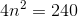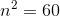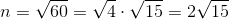# ISEE Upper Level Math : How to find the length of an edge of a tetrahedron

## Example Questions

### Example Question #1 : Tetrahedrons

A triangular pyramid, or tetrahedron, with volume 100 has four vertices with Cartesian coordinates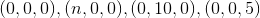where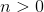.

Evaluate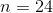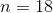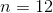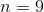Explanation:

The tetrahedron is as follows (figure not to scale):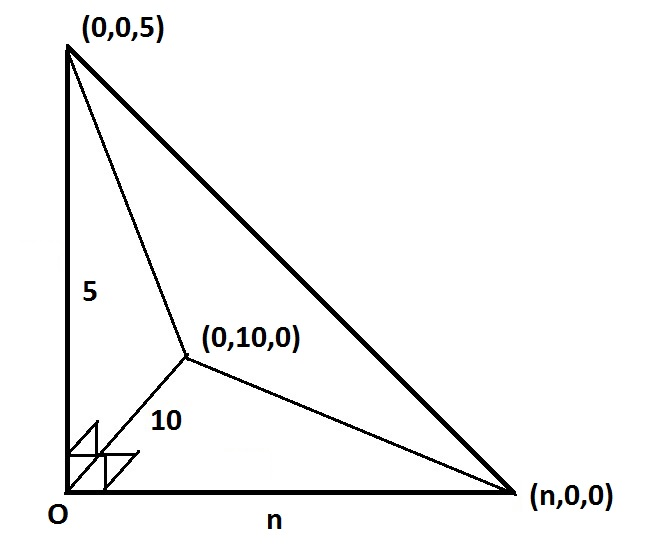This is a triangular pyramid with a right triangle with legs 10 andas its base; the area of the base is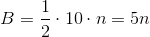The height of the pyramid is 5, so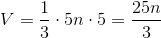Set this equal to 100 to get: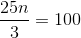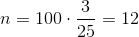### Example Question #2 : Tetrahedrons

A triangular pyramid, or tetrahedron, with volume 1,000 has four vertices with Cartesian coordinates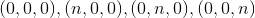where.

Evaluate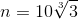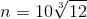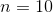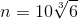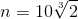Explanation:

The tetrahedron is as follows: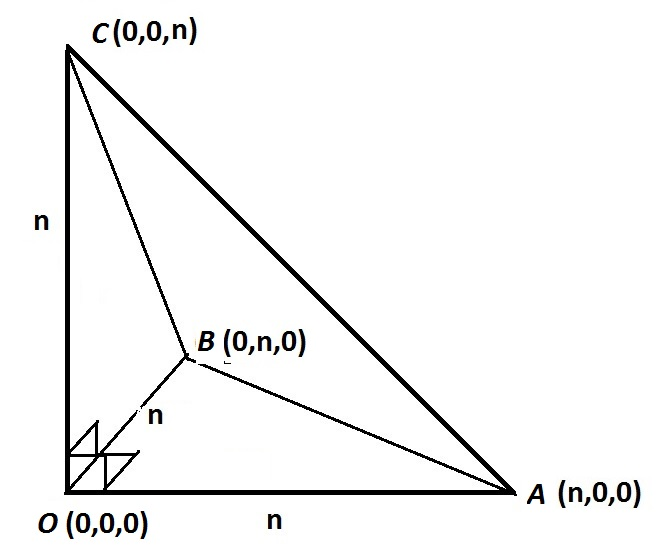This is a triangular pyramid with a right triangle with two legs of measureas its base; the area of the base is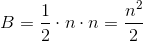Since the height of the pyramid is also, the volume is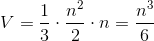.

Set this equal to 1,000: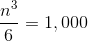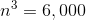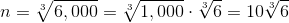### Example Question #3 : Tetrahedrons

A triangular pyramid, or tetrahedron, with volume 240 has four vertices with Cartesian coordinates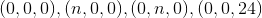where.

Evaluate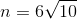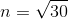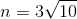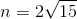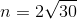Explanation:

The tetrahedron is as follows (figure not to scale):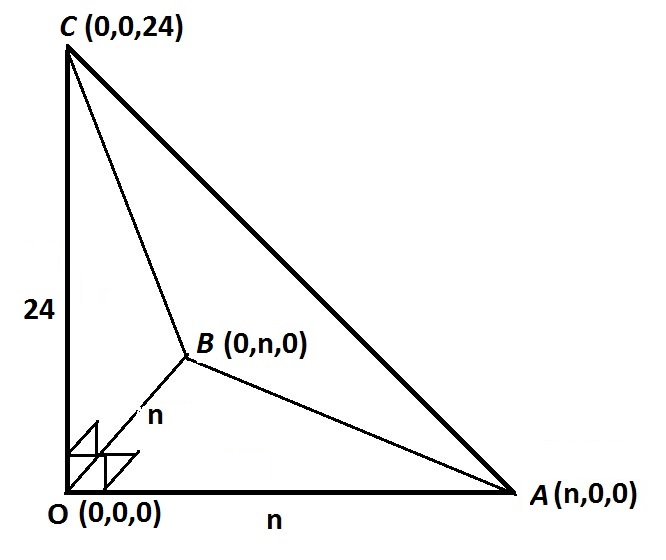This is a triangular pyramid with a right triangle with two legs of measureas its base; the area of the base isThe height of the pyramid is 24, so the volume is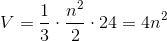Set this equal to 240 to get: